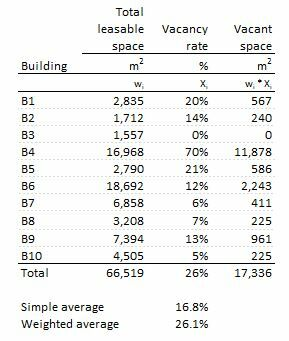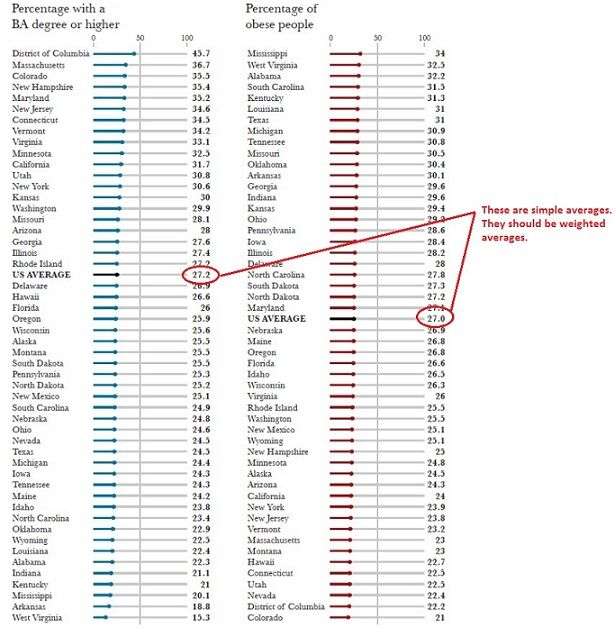• WORK
• TOOLS
• TRAINING
• VIEWS
• CONTACT

Views > Business modeling > Don't average averages

"Differing but in degree, of kind the same."

It’s useful to distinguish two types of average measures: Simple average (or arithmetic average) and weighted average. Both—simple and weighted averages—are widely used in practice but each type is more appropriate to use than the other for certain purposes and applications. Here, in the remainder of this blog, I’ll develop your understanding of each of these two types of average measures in depth—with particular emphasis on investments, market analysis and social sciences.

# DON'T AVERAGE AVERAGES

DON'T AVERAGE AVERAGESSIMPLE AVERAGE (ARITHMETIC AVERAGE)
The simple average of a set of observations is computed as the sum of the individual observations divided by the number of observations in the set. For example, assume there are five students in a small class with the following scores on a certain test—say math—82, 78, 83, 91 and 85. The teacher is interested in calculating their average score. Then the simple average would be 83.8 and is calculated as:

SA = (82 + 78 + 83 + 91 + 85) / 5 = 83.8.

The general formula for calculating the simple average can be written as:

This measure of average is useful if the teacher is interested in comparing the test performance of two different class of students—or the same class at different times. In calculating the simple average of a data set, however, it’s implicitly assumed that each data value carries the same weight. There’s no reason to assume otherwise for the average score example given above. But, if for some reason certain data values are more important than others—as we will see in the coming examples—different weights can be assigned to the values in the calculation of the average.

WEIGHTED AVERAGE
This leads us to the second type of average measures—the weighted average. Unlike the simple average, the weighted average is influenced by the weights given to the data values. For example, assume that the math scores listed above were the final grades of each student calculated by averaging the results of 5 different exams—three regular exams, a midterm, and a final exam—done during the semester. However, the professor wanted the midterm and final exams to contribute more than others to the final grade. Say the three regular exams each account for 15% of the grade, the midterm accounts for 25%, and the final accounts for 30%. Thus, the weights for the five exams are: 0.15, 0.15, 0.15, 0.25, and 0.3 respectively. One student achieves the following scores during the semester: 75, 80, 85, 76, and 90. This person’s final average score using the weighted average is:

WA = (0.15 * 75 + 0.15 * 80 + 0.15 * 85 + 0.25 * 76 + 0.3 * 90) / (0.15 + 0.15 + 0.15 + 0.25 + 0.3) = 82.0 / 1 = 82.

The general formula for calculating the weighted average can be written as:

INVESTMENT PERFORMANCE
Suppose an investor placed \$5 million into three investment funds at the beginning of the year. All investment earnings were reinvested. One million dollars were invested in a commercial real estate fund and produced a performance of 6.91% by the end of the year. Another three million dollars were invested in a common stock fund and produced a total return of 12.72%. The remaining one million dollars were invested in T-Bills and produced a return of 3.30% by year end.

Using the simple average to calculate the total return produces a misleading measure of financial performance. If we simply add up the three percentage gains and divide them by three that would leave us with a simple average of 7.64% on the rates of return. That doesn't take into account the fact that common stock accounted for 60% of the entire portfolio, while real estate and T-Bills combined accounted for the other 40%. The real performance of this portfolio is 9.76% and is calculated as follows:

WA = (1,000,000 * 6.91% + 3,000,000 * 12.72% + 1,000,000 * 3.3%) / (1,000,000 + 3,000,000 + 1,000,000) = (69,100 + 381,600 + 33,000) / 5,000,000 = 483,700 / 5,000,000 = 9.67%.

That’s 2.03 percentage points more than the simple average—a big difference.

MARKET ANALYSIS
Another example is the estimation of vacancy rates for certain building types—offices, shopping centers, apartments, etc.—within a specified real estate submarket. Vacancy rates measure the number of units—or the amount of square footage—that are available for occupancy at a point in time. It refers to the percentage of the stock of built space in the market that is not currently occupied. High vacancy rates are characteristics of oversupplied markets. Conversely, low vacancy rates signal that the demand for space exceeds supply of space and that there may be a need to add more space to the market.

To compute vacancy rates one must know the total stock of space in the market and the amount of space that is currently vacant. Once this building-specific information is known, the vacancy rate can be calculated as follows:

Let’s use the information below about individual buildings to calculate the vacancy rate for a given submarket. The submarket under investigation has 10 buildings—say 10 office buildings—with a total leasable space of 66,519m². Each building has a different vacancy rate. By simply averaging the individual building vacancy rates—column Xi in the table—we underestimate the vacancy rate of the submarket. The simple average of 16.8% disregards that buildings vary greatly in size. The weighted average on the other hand is heavily influenced by building B4—which is 70% vacant—yet represents a quarter of all the leasable space. The weighted average vacancy rate is equal to the total vacant space of 17,366m² divided by the total leasable space of 66,519m²—or 17,366 / 66,519 = 26.1%. Again, 26.1% versus 16.80%—a big underestimation.

SOCIAL SCIENCES
Social sciences offer fertile ground for confusing simple average for weighted average and vice versa. I see this happen a lot. Fertility rates, obesity rates, and educational attainment are just a sample of many examples where things could go wrong. Imagine that country A has 50 million women and country B has 1 million women. The fertility rate in country A is 1.1 while that of country B is 6.5. A’s average is 1.1, B’s is 6.5—but overall average isn’t 3.8 (the average of 1.1 and 6.5). It’s 1.2, the weighted average (50 * 1.1 + 1 * 6.5) / (50 + 1) = 61.5 / 51 = 1.21.

Here below are two examples taken from the book written by Alberto Cairo and entitled The Functional Art. The author used simple averages where weighted averages should have been used.Source: “The Functional Art”, Alberto Cairo.

Source: “The Functional Art”, Alberto Cairo.
Note: (*) I asked Alberto Cairo to share the raw data he used to calculate the world average fertility rate (black line) and the source for Figure 1.6—but Alberto never replied to my request. My suspicion grew from the discrepancy between his numbers and the UN data—the data source for his analysis.